Quantity: 0

Total: 0,00

0

# Cube puzzle

### Cube puzzle

Building cubes shown in several views from the available unit cubes aids spatial vision and other skills.

Mathematics

Keywords

cube puzzle, 3D puzzle, cube, view, front view, representation, view from the back, game, solid geometry, mathematics

### Related items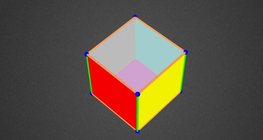#### Coloring a cube

Coloring the vertices, edges and faces of a given cube according to the criteria specified in the exercise.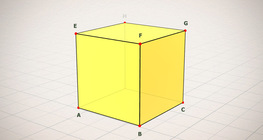#### Cube (exercises)

Edges, diagonals and faces of a cube can be identified by its vertices.#### Cube of cubes

An exercise about the regular hexahedron built from unit cubes to help deepen your knowledge of cubes.#### Cube sections (exercise)

Examining solids formed by the intersection of a cube and a plane.#### Net of a cube (exercises)

Not all nets consisting of 6 congruent squares are foldable into cubes.#### Building shapes (multi color)

Build 3D shapes from unit cubes with the help of several views.#### Building shapes (one color)

Build 3D shapes from unit cubes with the help of several views.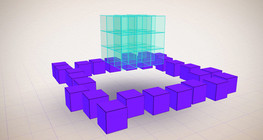#### Constructing shapes (3D)

Building 3D shapes into a given cube mesh with the help of several views.#### Cuboid

A cuboid is a polyhedron with six rectangular faces.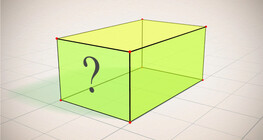#### Cuboid (exercises)

Edges, diagonals and faces of a cuboid can be identified by its vertices.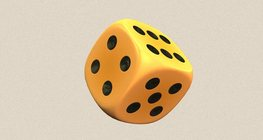#### Dice

Regular dice can be used for solving statistical and probability exercises.#### Grouping of cuboids

This animation demonstrates various types of cuboids through everyday objects.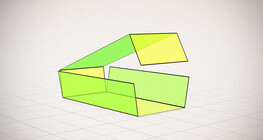#### Nets of a cuboid (exercises)

This animation demonstrates the different nets of a cuboid and includes a game.#### Shape games

Find views and nets of three-dimensional shapes.#### Volume and surface area (exercise)

An exercise about the volume and surface area of solids generated from a ´base cube´.